# GSEB Solutions Class 11 Chemistry Chapter 1 Some Basic Concepts of Chemistry

Gujarat Board GSEB Textbook Solutions Class 11 Chemistry Chapter 1 Some Basic Concepts of Chemistry Textbook Questions and Answers.

## Gujarat Board Textbook Solutions Class 11 Chemistry Chapter 1 Some Basic Concepts of Chemistry

### GSEB Class 11 Chemistry Some Basic Concepts of Chemistry Text Book Questions and Answers

Question 1.
Calculate the molecular mass of the following :

1. H2O
2. CO2
3. CH4

Solution:
1. Molecular mass of H2O
= 2 × Atomic mass of H + Atomic mass of O = 2 × 1 + 16 = 18.0 a.m.u.

2. Molecular mass of CO2
= Atomic mass of C + 2 × Atomic mass of O = 12 + 2 × 16 = 44.0 a.m.u.

3. Molecular mass of CH4
= Atomic mass of C + 4 × Atomic mass of H = 12 + 4 × 1 = 12 + 4 = 16 a.m.u.

Question 2.
Calculate the mass percent of different elements present in sodium sulfate (Na2SO4).
Solution:
Molecular mass of Na2SO4
= 2 × At. mass of Na + At. mass of S + 4 × At. mass of O = 2 × 23 + 32 + 4 × 16 = 142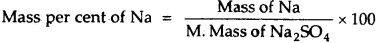$$\frac { 46 }{ 142 }$$ × 100 = 32.39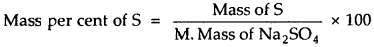$$\frac { 32 }{ 142 }$$ × 100 = 22.53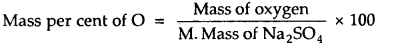$$\frac { 64 }{ 142 }$$ × 100 = 45.07Question 3.
Determine the empirical formula of an oxide of iron which has 69.9% iron and 30.1% dioxygen by mass.
Solution: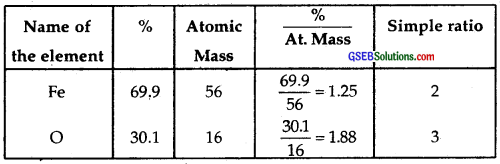The ratio between Fe and O is 2 : 3.
∴ The empirical formula = Fe2O3.

Question 4.
Calculate the amount of carbon dioxide that could be produced when.

1. 1 mole of carbon is burnt in the air
2. 1 mole of carbon is burnt in 16 g of dioxygen
3. 2 moles of carbon are burnt in 16 g of dioxygen.

Solution:
1.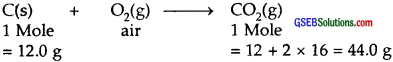Amount of CO2 produced = 44.0 g

2.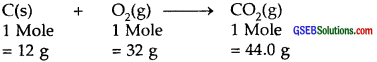32.0 g of O2 produce 44.0 g of CO2
16.0 g of O2 produce = $$\frac { 44 }{ 32 }$$ × 16 = 22.0 g of CO2
Amount of CO2 produced = 22.0 g

3. Amount of CO2 produced when 2 moles (= 24 g) of C are burnt in 16.0 g [limited amount] of O2 = 22.0 gQuestion 5.
Calculate the mass of sodium acetate (CH3COONa) required to make 500 mL of 0.375 molar aqueous solution. Molar mass of sodium acetate is 82.0245 g mol-1
Solution:
1000 mL of CH3COONa of 1 M solution contains 82.0245 g
500 mL of it 1 M solution contains = $$\frac { 82.0245 }{ 1000 }$$ × 500
500 mL of it of 0.375 M solution = $$\frac { 82.0245 }{ 1000 }$$ × 500 × 0.375 = 15.38 g.

Question 6.
Calculate the concentration of nitric acid in moles per liter in a sample that has a density, 1.41 g mL-1 and the mass percent of nitric acid in it being 69%.
Solution:
69% HNO3 by mass means that 69 g of HNO3 is present in 100 g of the solution.
Volume of 100 g of the solution =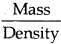= $$\frac { 100 }{ 1.41 }$$ = 70.92 mL
Molar mass of HNO3 = 63.0 g mol-1
∴ 69 g HNO3 = $$\frac { 69.0 }{ 63.0 }$$ moles = 1.095 moles
Thus 70.92 mL of solution contains HNO3 = 1.095 moles
∴ 1000 mL (= 1 L) solution contains HNO3 = $$\frac { 1.095 }{ 70.92 }$$ × 1000
∴ Concentration of nitric acid = 15.44 mol L-1.Question 7.
How much copper can be obtained from 100 g of copper sulfate (CuSO4)?
Solution:
Molar mass of CuSO4 = Atomic mass of Cu + Atomic mass of S + 4 × Atomic mass of O
= 63.5 + 32.0 + 4 × 16.0 = 159.5 g mol-1
159.5 g of CuSO4 = 63.5 g of Cu
100 g of CuSO4 = $$\frac { 63.5 }{ 159.5 }$$ × 100 = 39.81 g
∴ Mass of Cu that can be obtained = 39.81 g.

Question 8.
Determine the molecular formula of an oxide of iron in which the mass percent of iron and oxygen are 69.9 and 30.1 respectively.
Solution:
Step I: To calculate the empirical formula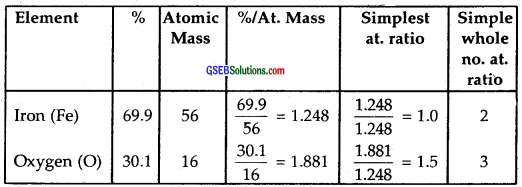Hence the empirical formula of an oxide of Iron = Fe2O3.

Step II: The molecular formula of the oxide of iron is the same as the empirical formula, i.e., Fe2O3.
Note: Molecular formula can be determined if the molecular mass of oxide of iron is given. It is not given in the question.Question 9.
Calculate the atomic mass (average) of chlorine using the following data :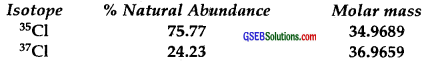Solution:
The average atomic mass of chlorine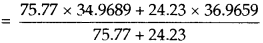$$\frac { 3545277 }{ 100 }$$ = 35.45

Question 10.
In three moles of ethane (C2H6), calculate the following:

1. number of moles of carbon atoms
2. no. of moles of hydrogen atoms
3. no. of molecules of ethane.

Solution:
1. 1 Mole of ethane (C2H6) contains 2 moles of carbon atoms
∴ 3 moles of C2H6 will contain 6 moles of carbon (C) atoms.

2. 1 mole of C2H6 contains 6 moles of hydrogen (H) atoms
∴ 3 moles of C2H6 will contain 3 × 6 = 18 moles of H atoms.

3. 1 mole of C2H6 contains 6.023 × 1023 molecules of ethane
∴ 3 moles of C2H6 will contain 3 × 6.023 × 1023
= 1.8069 × 1024 molecules.Question 11.
What is the concentration of sugar (C12H22O11) in mol L-1 if its 20 g are dissolved in enough water to make a final volume up to 2 L?
Solution:
Molar mass of sugar = 12 × 12 + 22 × 1 × 11 × 16 = 342 g mol-1
No. of moles of sugar in 20 g = $$\frac { 20 }{ 342 }$$
Concentration in mol L-1 = No. of moles per litre of the solution
= $$\frac{20}{342 \times 2}$$ [∵ Vol. of solution = 2 L]
= 0.029 mol L-1

Question 12.
If the density of methanol is 0.793 kg L-1 h What is its volume needed for making 2.5 L of its 0.25 M solution ?
Solution:
Molar mass of methanol (CH3OH) = 1 × 12 + 4 × 1 + 1 × 16 = 32 g mol-1
Moles of methanol in 2.5 L of its 0.25 M solution = 2.5 × 0.25 = 0.625 mole
mole Mass of methanol = 32 × 0.625 g = 20.0 g
Density of methanol = 0.793 kg L-1
∴ Volume of methanol required = $$\frac { 20.0 }{ 793 }$$ – 0.025 LQuestion 13.
The pressure is determined as force per unit area of the surface. The SI unit of pressure, pascal is as shown below :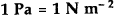If the mass of air at sea level is 1034 g cm-2, calculate the pressure in the pascal.
Solution:
Acceleration due to gravity (g) = 9.806 ms-2
Mass of air = 1.034 kg cm-2 =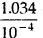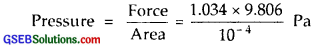= 10.138 × 104 Pa
The pressure of air at sea level = 1.0138 × 105 pa.

Question 14.
What is the SI unit of mass? How is it defined?
Solution:
SI unit of mass is Kg (kilogram) It is defined as the mass of platinum-iridium (Pt-Ir) cylinder that is stored in an airtight jar at the International Bureau of Weights and Measures in France.Question 15.
Match the following prefixes with their multiples.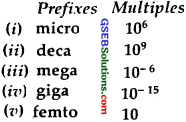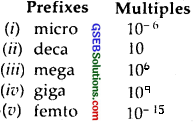Question 16.
What do you mean by significant figures?
Solution:
Significant figures refer to the number of digits in a number that has some importance in the magnitude of a given number.
Example: 30.4560
n is equal to 5.
Example: 16708.4300
n is equal to 7.

Question 17.
A sample of drinking water was found to be severely contaminated with chloroform, CHCl3, supposed to be carcinogenic in nature. The level of contamination was 15 ppm (by mass)

1. Express this in percent by mass.
2. Determine the molality of chloroform in the water sample.

Solution:
1. 106 gm of solution contains 15 g of CHCT3
1 gm of solution contains =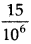100 gm of solution contains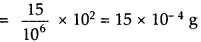∴ percent by mass = ~ 15 × 10-4g.

2. Molafity of CHCl3 : 106 gm of the solution contains 15 g of CHCl3
∴ Wt. of water 1000000 – 15 = 999985 gm
Now, 999985 g of water contains 15 g of CHCl3
1000 g of water contains = $$\frac { 15 }{ 999985 }$$ × 1000 g of CHCl3
Molar mass of CHCl3 = 12 + 1 + 3 × 35.5 = 119.5 g mol-1
∴ Monality = $$\frac { 15 }{ 999985 }$$ × $$\frac { 1000 }{ 119.5 }$$m = 1.25 × 10-4m.Question 18.
Express the following in the scientific notation

1. 0.0048
2. 234,000
3. 8008
4. 500.0
5. 6.0012

Solution:

1. 0.0048 =$$\frac { 48 }{ 10000 }$$= 48 × 10-4 = 4.8 × 10-3
2. 234,000 = 2.34 × 105
3. 8008 = 8.008 × 103
4. 500.0 = 5.00 × 103
5. 6.0012 = 6.0012 × 10°.

Question 19.
How many significant figures are present in the following?

1. 0.0025
2. 208
3. 5005
4. 126,000
5. 500.0
6. 2.0034

Solution:

1. 2
2. 3
3. 4
4. 6
5. 4
6. 5

Question 20.
Round up the following up to three significant figures :

1. 34.216
2. 10.4107
3. 0.04597
4. 280.8

Solution:

1. 34.126 ~ 34.2
2. 10.4107 ~ 10.4
3. 0.04597 ~ 0.0460
4. 280.8 ~ 281Question 21.
The following data are obtained when dinitrogen and dioxygen react together to form different compounds :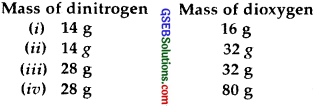(1) Which law of chemical combination is obeyed by the above experimental data? Give its statement.
(2) Fill in the blanks in the following conversion :

1. 1 km = _______ mm = _______ pm
2. 1 mg = _______ kg = _______ ng
3. 1 mL = _______ L = _______ dm3

Solution:
(1) The given data is in accordance with the law of multiple proportions, which states; When two elements combine to form two or more than two compounds, the weights of one of the two elements which combine with the fixed weight of the order bears a simple ratio to one another.

In the said question, if we fix the weight of dinitrogen at 14 g, then the weights of dioxygen which combines with the fixed weight (=14 g) of dinitrogen will be 16, 32, 16, 40 which are in the simple whole-number ratio of 1: 2: 1: 2.5 or 2: 4: 2: 5.

(2)

1. 1 km = 106 mm = 1015 pm
2. 1 mg = 10-6 kg = 106 ng
3. 1 mL = 10-3 L = 10-3 dm3

Question 22.
If the speed of light is 3.0 × 108 ms-1, calculate the distance covered by light in 2.00 ns.
Solution:
Distance covered by light in one second = 3.0 × 108 m
∴ Distance covered by light in 2.00 ns or 2 × 10-9 second = 3.0 × 108 × 2 × 10-9 m
∴ Distance covered in 2.00 ns = 6.0 × 10-1 m = 0.6 m.QUestion 23.
In a reaction,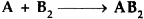Identify the limiting reagent, if any, in the following reaction mixtures :

1. 300 atoms of A + 200 molecules of B
2. 2 mol A + 3 mol B
3. 100 atoms of A + 100 molecules of B
4. 5 mol A + 2.5 mol B
5. 2.5 mol A + 5 mol B.

Solution:

1. The given reaction is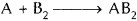Here 300-atoms of A requires 300 molecules of B. Since there are only 200 molecules of B provide.
∴ B is the limiting reagent.
2. 3 mol B requires 2 mol A. Since only 2 mol of A is provided, therefore A is the limiting reagent.
3. 100 atoms of A + 100 molecules of B constitute a stoichiometric mixture. Neither A nor B is the limiting reagent.
4. B is the limiting reagent as 5 mol A requires 5 mol B but only 2.5 mol B is given.
5. A is the limiting reagent as 5 mol B requires 5 mol A, but only 2.5 mol A are provided.

Question 24.
Dinitrogen and dihydrogen react with each other to produce ammonia according to the following chemical equation :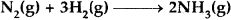1. Calculate the mass of ammonia produced if 2.00 × 103 g dinitrogen reacts with 1.00 × 103 g of dihydrogen.
2. Will any of the two reactants remain unreacted?
3. If yes, which one and what would be its mass?

Solution: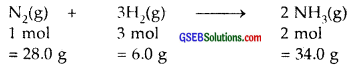1.  28.0 g of N2 require 6.0 g of H2 to produce 34.0 g of NH3
2.00 × 103 g of N2 will produce $$\frac { 34 }{ 28 }$$ × 2.00 × 103 g of NH3
= 2.43 × 103 g NH3 = 2430 g NH3

2.  Yes, Dihydrogen will remain unreacted to some extent

3.  Amount of hydrogen that remains unreacted 28.0 g of N2 require 6.0 g of H2
200 × 103 g of N2 will require $$\frac { 6.0 }{ 28.0 }$$ × 2.00 × 103 of H2 = 428.5 g of H2
∴ Amount of hydrogen = (1.00 × 103 – 428.5) g
that remains unreacted = 571.5 gQuestion 25.
How are 0.50 mol Na2CO3 and 0.50 M Na2CO3 different?
Solution:
0.50 mol Na2CO3 means $$\frac { 1 }{ 2 }$$ the molar mass of Na2CO3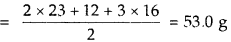∴ 0.50 mol Na2CO3 represents the mass = 53.0 g of it whereas 0.50 M Na2CO3 represents its molarity in solution 0.50 M Na2CO3 indicates that 53.0 g of Na2CO3 have been dissolved in 1 L of its solution.

Question 26.
If ten volumes of dihydrogen gas react with five volumes of dioxygen gas, how many volumes of water vapor would be produced?
Solution: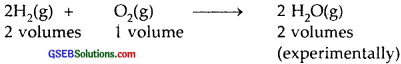∴ The ratio by volumes is 2: 1: 2
∴ 10 volumes of dihydrogen will react with 5 volumes of dioxygen to produce 10 volumes of water vapors.

Question 27.
Convert the following into basic units :

1. 28.7 pm
2. 1515 ps
3. 25365 mg

Solution:

1. 28.7 pm = 2.87 x 10-11 m
2. 15.15 ps = 15.15 x 10-6 s ∼ 1.515 x 10-5 s
3. 25365 mg = 2.5365 x 10-2 kg.

Question 28.
Which one of the following will have the largest number of atoms?

1. 1 g Au(s)
2. 1 g Na(s)
3. 1 g Li(s)
4. 1 g Cl2(g)

Solution:
1. 1 gm atom of Au(s) = 197 g have 6.023 × 1023 atoms of Au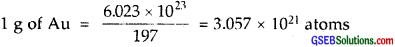2. 23.0 g of Na = 6.023 × 1023 atoms of Na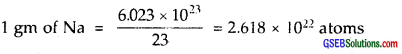3. 7.0 g of Li = 6.023 × 1023 atoms of Li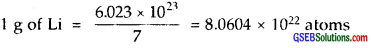4. 71.0 g of Cl2 = 2 × 6.023 × 1023 atoms of Cl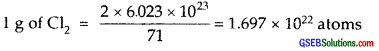By comparison (iii) i.e., 1 g Li(s) will have max. no. of atoms, viz., 8.604 × 1022 atoms.

Question 29.
Calculate the molarity of a solution of ethanol in water in which the mole fraction of ethanol is 0.040?
Solution:
Let us calculate the mass of ethanol in which its mole fraction is 0.040.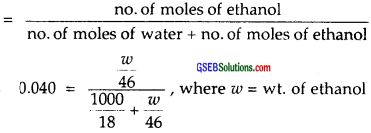Solving for ω = wt. of ethanol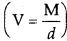density is 1 gm cm-3 = 106.48 g
∴ Molarity of the solution = $$\frac { 106.48 }{ 46 }$$ = 2.315 M.

Question 30.
What will be the mass of one 12C atom in g?
Solution:
We want to calculate the mass of one atom of 12C.
1 gm atom of 12C = 12.0 g
6.23 × 1023 atoms of 12Carbon weigh = 12.0 g
1 atom of 12Carbon weight =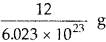∴ wt. of 1 atom of 12C = 1.99265 × 10-23 g.

Question 31.
How many significant figures should be present in the answer to the following calculations?

1.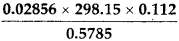2.  5 × 5.364
3. 0.0125 + 0.7864 + 0.0215

Solution:

(1)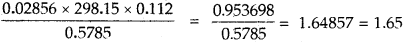It should have 3 significant figures.

(2) 5 × 5.364 = 26.82
It should have 4 significant figures.

(3) 0. 0215 + 0.7864 + 0.0215 = 0.8204
It should have 4 significant figures.

Question 32.
Use the data given in the following table to calculate the molar mass of naturally occurring argon isotopes :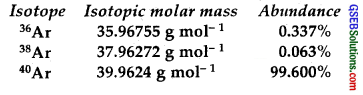Solution:
The molar mass of naturally occurring Argon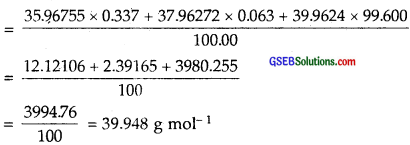Question 33.
Calculate the number of atoms in each of the following :

1. 52 moles of Ar
2. 52 u of He
3. 52 g of He.

Solution:
(1) 1 mole of Argon (Ar) contains 6.023 × 1023 atoms
52 moles of Ar contains 6.023 × 1023 × 52 atoms = 3.13 × 1025 atoms

(2) 4 u of Helium (He) = 1 atom of He
52 of He = $$\frac { 1 }{ 4 }$$ × 52 = 13 atoms

(3) 4 g of He contains = 6.023 × 1023 atoms
52 g of He contains =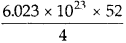= 7.8286 × 1024 atoms.

Question 34.
A welding fuel gas contains carbon and hydrogen only. Burning a small sample of it in oxygen gives 3.38 g carbon dioxide, 0.690 g of water, and no other products. A volume of 10.0 L (measured at STP) of this welding gas is found to weigh 11.6 g. Calculate

1. empirical formula
2. the molar mass of the gas, and
3. molecular formula.

Solution:
Welding fuel gas is made up of C and H only C×Hy. 10.0 L of this gas at STP weighs = 11.6 g
22.4 L of this gas at STP weights = $$\frac { 11.6 }{ 10.0 }$$ × 22.4 g = 25.98
Since 22.4 L of any gas at STP weighs = Molecular mass
∴ Molar mass of the welding fuel gas = 26.0 (near to whole no.)
∴ It must contain 2 atoms of C and 2 atoms of hydrogen
∴ Its molecular formula is C2H2 [x = y = 2]
Its empirical formula is CH.Question 35.
Calcium carbonate reacts with aqueous HCl to give CaCl2 and CO2 according to the reaction,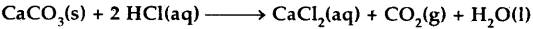What mass of CaCO3 is required to react completely with 25 mL of 0.75 M HCl?
Solution:
The given reaction is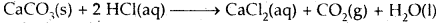Let us find out the weight of HCl present in 25 mL of 0.75 M HCl 1000 mL of 1.0 M HCl contains = 36.5 g of it
1000 mL of 1.0 M HCl contains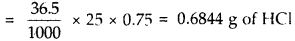According to the equation;
73 g of HCl [2(1 + 35.5)] reacts with 100.0 g of CaCO3
0.6844 g of HCI reacts with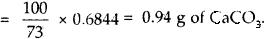Question 36.
Chlorine is prepared in the laboratory by treating manganese dioxide (MnO2) with aqueous hydrochloric acid according to the reaction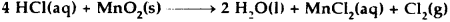How many grams of HCl react with 5.0 g of manganese dioxide?
Solution:
The chemical equation is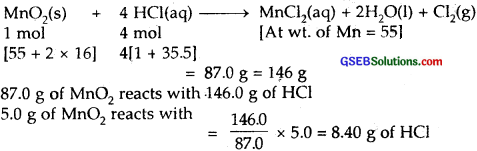∴ Amount of HCl in grams which will react with 5.0 g of manganese dioxide = 8.40 g.